$$\hspace{5cm}$$

Welcome to BHMSMAfMRI, an R package to analyze functional MRI (fMRI) data, or other multiscale data. This manual shows how the BHMSMAfMRI package functions are used to analyze fMRI data and should be helpful for the first-time user. In Section @ref(secintro), we give a short introduction and non-mathematical overview of the methodology, and in Section @ref(secuse), we discuss the package functions in a systematic way and apply them to analyze a simulated fMRI dataset.

## Introduction and overview

The BHMSMAfMRI package performs Bayesian hierarchical multi-subject multiscale analysis (BHMSMA) of fMRI data (Sanyal and Ferreira 2012), or other multiscale data. Though fMRI data is generally 3D, currently BHMSMAfMRI considers analysis of 2D slices only.

The main features of the BHMSMA method are that:

• it takes into account both the temporal and the spatial information contained in the fMRI data,
• it performs multi-subject analysis and borrows strength across subjects for precise estimation of the effect sizes (i.e., brain activations), and provides a straightforward way to obtain group activation map, and
• it does not use Markov Chain Monte Carlo (MCMC) simulation and is fast.

BHMSMA models the temporal variation present in the fMRI data through a general linear model (GLM) and then considers discrete wavelet transform of the standardized regression coefficients for harnessing the spatial information. In the wavelet domain, each wavelet coefficient is assigned a mixture prior that is a combination of a Gaussian density and a point mass at zero. This prior specification takes into account the sparsity of the wavelet coefficients. For the mixture probabilities BHMSMA considers a prior that depends on few hyperparameters. Inference is carried out by an empirical Bayes methodology without using MCMC methods. The inference uses approximation of one-dimensional integrals only. The posterior mean of the regression coefficients are obtained by using the posterior mean of the wavelet coefficients in the inverse discrete wavelet transform. Further, the posterior wavelet coefficients are averaged over subjects and used in the inverse discrete wavelet transform to obtain posterior group estimate of the regression coefficients. Posterior uncertainty is assessed based on simulations from the posterior distribution of the regression coefficients.

The BHMSMAfMRI package fits the BHMSMA model to the fMRI data and provides estimates of the hyperparameters along with their standard error, posterior mean of the wavelet coefficients, posterior mean of the regression coefficients, samples from the posterior distribution of the regression coefficients and posterior group estimate of the regression coefficients. The posterior samples can be used to compute the estimates of posterior standard deviation and posterior probability maps.

## Use of package functions with examples

In this section we illustrate the use of the package functions. We assume that prior to applying our methodology, the fMRI data have been preprocessed for necessary corrections like realignment or motion correction, slice-timing correction, coregistration with anatomical image and normalization. However, the data must not be spatially filtered before applying BHMSMA, because our approach is to include the spatial information into modeling instead of filtering it out. Preprocessing can be perfomed by using available softwares/packages like SPM (Friston et al. 2007), BrainVoyager (Goebel, Esposito, and Formisano 2006), AFNI (Cox 1996), and FSL (Smith et al. 2004). In the following subsections, we show the use of the package functions in a systematic way.

### The main function BHMSMA

BHMSMA is the main function of the BHMSMAfMRI package. BHMSMA accepts fMRI data as a 4D array. The data can be imported from various image files by the readfmridata function. BHMSMA successively calls the following functions to perform the whole analysis — glmcoef to obtain regression coefficient map of the regressor of interest, waveletcoef to perform 2D wavelet transformation of the regression coefficient map, hyperparamest to obtain estimates of the BHMSMA model hyperparameters, postmixprob to obtain estimates of the mixture probabilities that define posterior distribution of the wavelet coefficients, postwaveletcoef to obtain the posterior estimates of the wavelet coefficients and finally postglmcoef to obtain the posterior smoothed version of the regression coefficient map. If true coefficients are given, BHMSMA also returns mean squared error (MSE) estimates. Here is a quick look at the usage and outputs of the function.

library(BHMSMAfMRI)
BHMSMAmulti <- BHMSMA(n, grid, data, designmat, k, "multi", truecoef)
names(BHMSMAmulti)
 "GLMCoefStandardized"      "GLMCoefSE"
 "WaveletCoefficientMatrix" "hyperparam"
 "hyperparamVar"            "posteriorMixProb"
 "Waveletcoefposterior"     "GLMcoefposterior"

The dimension of data is c(n,grid,grid,ntime) where n is the number of sujects, grid^2 is the total number of voxels in the data and ntime is the length of time-series for each voxel. The argument k selects the regressor of interest from the design matrix designmat which can have multiple regressor columns. Following sections break down the whole analysis shown above by showing specific uses and outputs of all the above functions and several others of the BHMSMAfMRI package.

### Reading fMRI Data: readfmridata

The function readfmridata can read fMRI data file(s) stored in ANALYZE format (.img/.hdr files), NIFTI format (.img/.hdr files or .nii files) or AFNI format (.BRIK/.HEAD files). The reading of the fMRI data files is done using R package oro.nifti (Whitcher, Schmid, and Thornton 2011), which is loaded when BHMSMAfMRI package is loaded.

For illustration, we consider a simulated fMRI dataset stored in ANALYZE format and provided within the BHMSMAfMRI package. The dataset contains noisy fMRI data collected over a $$32 \times 32$$ grid of a single axial brain slice and at 9 timepoints for 3 subjects. The following code illustrates how the function readfmridata can be used to import the data from the image files. The simulated dataset is extracted in the directory fmridata within the R temporary folder.

library(BHMSMAfMRI)
##
##  Welcome! Thanks for trying BHMSMAfMRI.
##
##  Website: https://nilotpalsanyal.github.io/BHMSMAfMRI/
##  Bug report: https://github.com/nilotpalsanyal/BHMSMAfMRI/issues
fpath <- system.file("extdata", package="BHMSMAfMRI")
untar(paste0(fpath,"/fmridata.tar"), exdir=tempdir())
n <- 3
grid <- 32
ntime <- 9
data <- array(dim=c(n,grid,grid,ntime))
for(subject in 1:n)
{
directory <- paste0(tempdir(),"/fmridata","/s0",subject,"/")
prefix=paste0("s0",subject,"_t"), nimages=ntime, dim.image=c(grid,grid,1))
data[subject,,,] <- a[,,1,]
}
dim(a)
##  32 32  1  9

The above code reads all the data files for all subjects into a 4D array data. For each subject, the data were generated by adding Gaussian random noise to the true regression coefficient image with activation in 3 regions. The positions of 2 activation regions were varied across subject. The underlying design was a block design. The true regression coefficient images and the design matrix are also included in the package and can be read as follows.

data(fmridata)
names(fmridata)
##  "grid"         "nsubject"     "TrueCoeff"    "DesignMatrix"
truecoef <- fmridata$TrueCoeff designmat <- fmridata$DesignMatrix
dim(truecoef)
##   3 32 32
dim(designmat)
##  9 2

Now, we have truecoef which is an array of dimension (3, 32, 32) containing the true regression coefficients, data which is an array of dimension (3, 32, 32, 9) containing time series of noisy observations for all the subjects and designmat which is the design matrix used to generate the data. Note that, the R package neuRosim (Welvaert et al. 2011) can be used to generate fMRI data.

par(mfrow=c(1,n), cex=1)
for(subject in 1:n)
image(truecoef[subject,,], main=paste0("Subject ",subject),
col=heat.colors(8))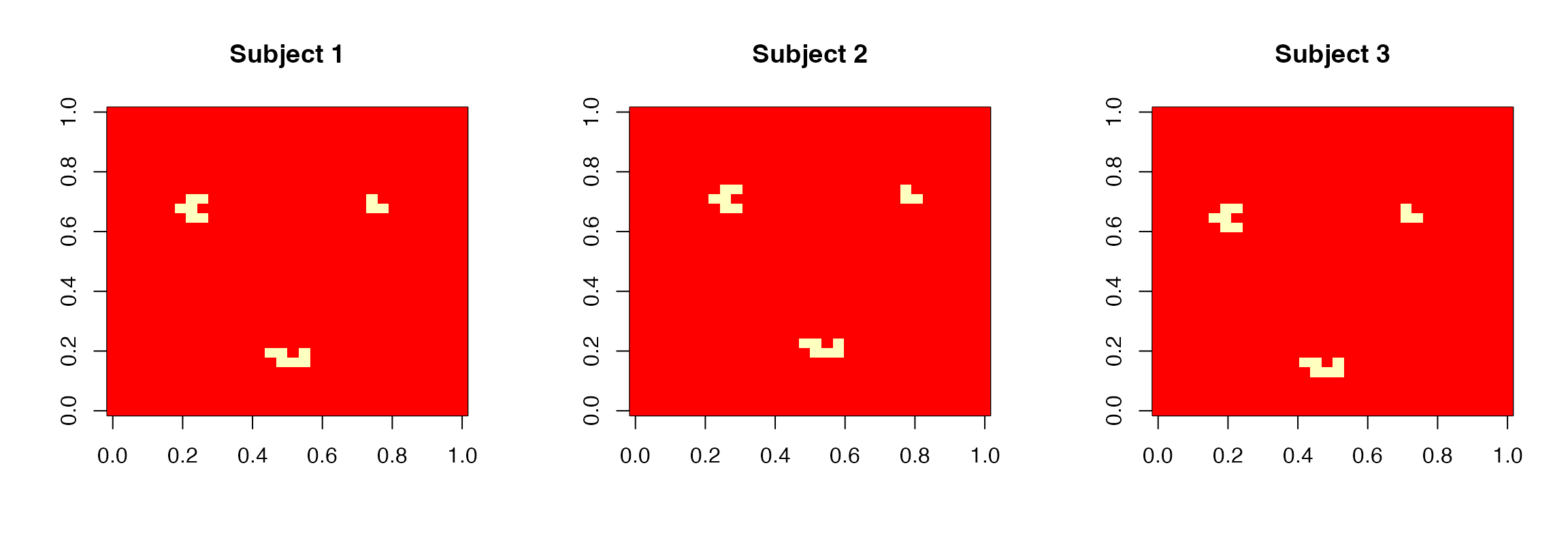True regression coefficient images for the 3 subjects

### Temporal modeling through GLM: glmcoef

Now, we fit a general linear model to the time series of each voxel and obtain the estimated regression coefficients for all the regressors included in designmat by using the function glmcoef as follows.

glmmap <- glmcoef(n, grid, data, designmat)
names(glmmap)
##  "GLMCoefStandardized" "GLMCoefSE"
dim(glmmap$GLMCoefStandardized) ##  3 32 32 2 dim(glmmap$GLMCoefSE)
##   3 32 32  2

The output glmmap contains the estimated standardized regression coefficients and their standard error estimates. From now on, we focus on the 2$$^\text{nd}$$ regressor as the regressor of interest. Figure @ref(fig:GLMCoef), obtained by the following code, shows the images of its standardized regression coefficients estimates for the 3 subjects.

k <- 2
par(mfrow=c(1,n), cex=1)
for(subject in 1:n)
image(abs(glmmap$GLMCoefStandardized[subject,,,k]), col=heat.colors(8), zlim=c(0,6), main=paste0("Subject ",subject))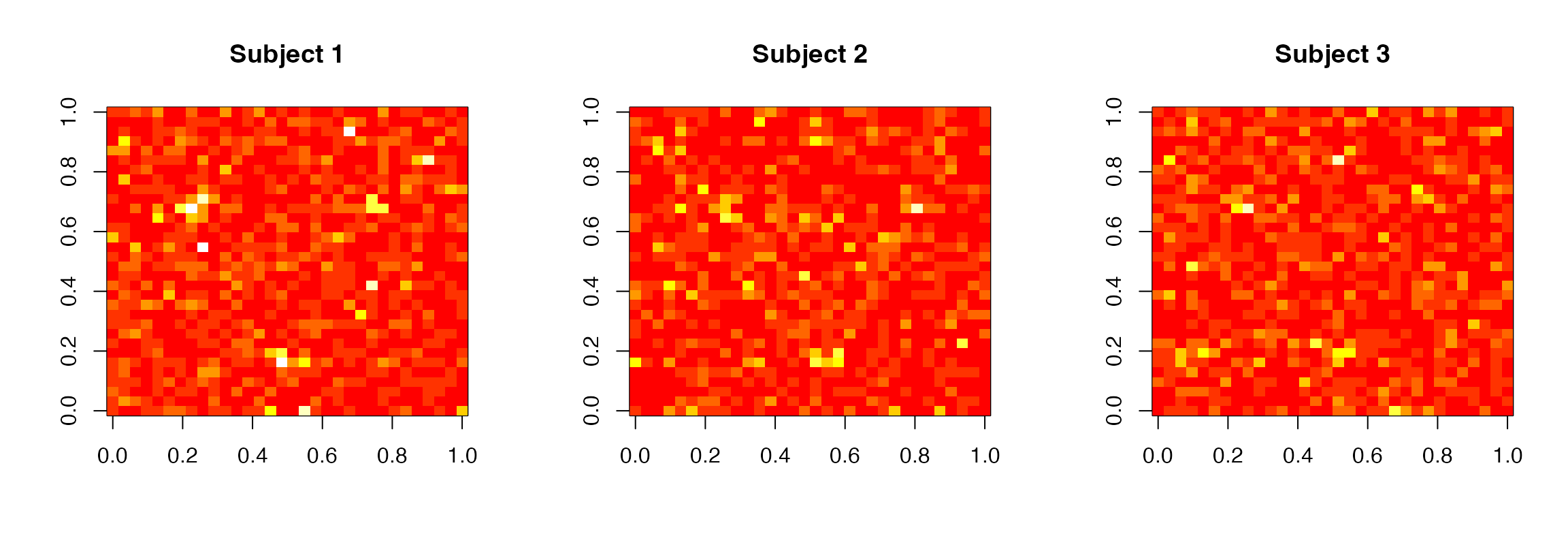Standardized regression coefficient estimates images for the second regressor for all subjects ### Wavelet transform of the GLM coefficients: waveletcoef Next, we apply the discrete wavelet transform to the standardized regression coefficient images of each subject. The wavelet transformation is performed by the using the R package wavethresh (Nason 2013), which is loaded when BHMSMAfMRI package is loaded. The function waveletcoef returns the wavelet coefficients for the selected regressor for all the subjects as a matrix. Below it is illustrated. wavecoefglmmap <- waveletcoef(n, grid, glmmap$GLMCoefStandardized[,,,k],
wave.family="DaubLeAsymm", filter.number=6, bc="periodic")
names(wavecoefglmmap)
##  "WaveletCoefficientMatrix"
dim(wavecoefglmmap$WaveletCoefficientMatrix) ##  3 1023 In the wavelet transform, the user can choose the wavelet family (one of DaubLeAsymm and DaubExPhase), the number of vanishing moments (filter.number) and the boundary condition (symmetric or periodic) to be applied. For fMRI data, we recommend the use of Daubechies least asymmetric wavelet transform (DaubLeAsymm) with 6 vanishing moments and periodic boundary condition. ### Estimating the model hyperparameters: hyperest The BHMSMA model has six hyperparameters, which are estimated by their maximum likelihood estimates (MLEs) following an empirical Bayes approach. We can estimate the hyperparameters by performing multi-subject analysis or single subject analysis. In multi-subject analysis, the likelihood function of the hyperparameters is constructed over all subjects and maximized to obtain their estimates. In single subject analysis, for each subject, separate likelihood function of the hyperparameters is constructed and maximized. Hence, for single subject analysis, for each subject we obtain a set of estimates of the hyperparameters. Clearly, multi-subject analysis benefits from being able to borrow strength across subjects and produces more precise estimates. The function hyperparamest computes the hyperparameter estimates and their standard error estimates. The type of analysis must be specified as analysis="multi" or "single". The following code illustrates the use of the function hyperparamest and the output. options(width = 100) hyperest <- hyperparamest(n, grid, wavecoefglmmap$WaveletCoefficientMatrix,
analysis = "multi")
names(hyperest)
##  "hyperparam"    "hyperparamVar"
round(hyperest$hyperparam,3) ##  1.295 1.193 0.617 0.814 2.242 0.204 signif(hyperest$hyperparamVar,4)
##            [,1]       [,2]       [,3]       [,4]       [,5]       [,6]
## [1,]  5.085e-19  2.002e-34 -5.642e-35 -1.241e-35  1.368e-34  5.295e-35
## [2,]  2.002e-34  4.319e-19 -1.040e-34  1.143e-35  4.097e-34  2.583e-35
## [3,] -5.642e-35 -1.040e-34  1.153e-19  5.909e-36 -8.143e-35 -3.115e-35
## [4,] -1.241e-35  1.143e-35  5.909e-36  2.008e-19 -6.876e-34  1.957e-35
## [5,]  1.368e-34  4.097e-34 -8.143e-35 -6.876e-34  1.525e-18 -5.394e-36
## [6,]  5.295e-35  2.583e-35 -3.115e-35  1.957e-35 -5.394e-36  1.265e-20

From the hyperparameter estimates, we can compute the estimates of $$a_{kl}$$, $$b_{kl}$$ and $$c_{kl}$$ (Sanyal and Ferreira 2012) for all levels as follows.

a.kl <- hyperest$hyperparam * 2^(-hyperest$hyperparam * (0:4))
b.kl <- hyperest$hyperparam * 2^(-hyperest$hyperparam * (0:4))
c.kl <- hyperest$hyperparam * 2^(-hyperest$hyperparam * (0:4))
round(a.kl,3)
##  1.295 0.566 0.248 0.108 0.047

### Computing posterior distribution of the wavelet coefficients: postmixprob, postwaveletcoef

Given the values of the hyperparameters, the marginal posterior distribution of the wavelet coefficients is a mixture of a Gaussian and a point mass at zero with mixture probabilities $$\bar{p}_{iklj}$$. BHMSMA computes $$\bar{p}_{iklj}$$ values using Newton-Cotes numerical integration method. The function postmixprob computes the values $$\bar{p}_{iklj}$$ for all subjects and returns in a matrix. The following code illustrates it.

pkljbar <- postmixprob(n, grid, wavecoefglmmap$WaveletCoefficientMatrix, hyperest$hyperparam, analysis = "multi")
names(pkljbar)
##  "pkljbar"
dim(pkljbar$pkljbar) ##  3 1023 round(pkljbar$pkljbar[1,1:10],4)
##   0.9868 0.9115 0.9466 0.9095 0.9174 0.9237 0.8922 0.8911 0.9998 0.9599

Once $$\bar{p}_{iklj}$$ values are obtained, the marginal posterior distribution of the wavelet coefficients are entirely known. With the hyperparameter estimates and the $$\bar{p}_{iklj}$$ values, the function postwaveletcoef computes the posterior mean and the posterior median of the wavelet coefficients. The following code illustrates it.

postwavecoefglmmap <- postwaveletcoef(n, grid,
wavecoefglmmap$WaveletCoefficientMatrix, hyperest$hyperparam, pkljbar$pkljbar, analysis = "multi") names(postwavecoefglmmap) ##  "PostMeanWaveletCoeff" "PostMedianWaveletCoeff" dim(postwavecoefglmmap$PostMeanWaveletCoef)
##     3 1023
dim(postwavecoefglmmap$PostMedianWaveletCoeff) ##  3 1023 ### Computing posterior mean of the regression coefficients: postglmcoef Given the posterior mean of the wavelet coefficients, the function postglmcoef is used to obtain the posterior means of the regression coefficients. The following code shows its use. postglmmap <- postglmcoef(n, grid, glmmap$GLMCoefStandardized[,,,k],
postwavecoefglmmap$PostMeanWaveletCoef, wave.family="DaubLeAsymm", filter.number=6, bc="periodic") str(postglmmap,vec.len = 3, digits.d = 2) ## List of 1 ##$ GLMcoefposterior: num [1:3, 1:32, 1:32] -0.36 -0.188 -0.014 0.231 ...
par(mfrow=c(1,n), cex=1)
for(subject in 1:n)
image(abs(postglmmap$GLMcoefposterior[subject,,]), col=heat.colors(8), zlim=c(0,6), main=paste0("Subject ",subject))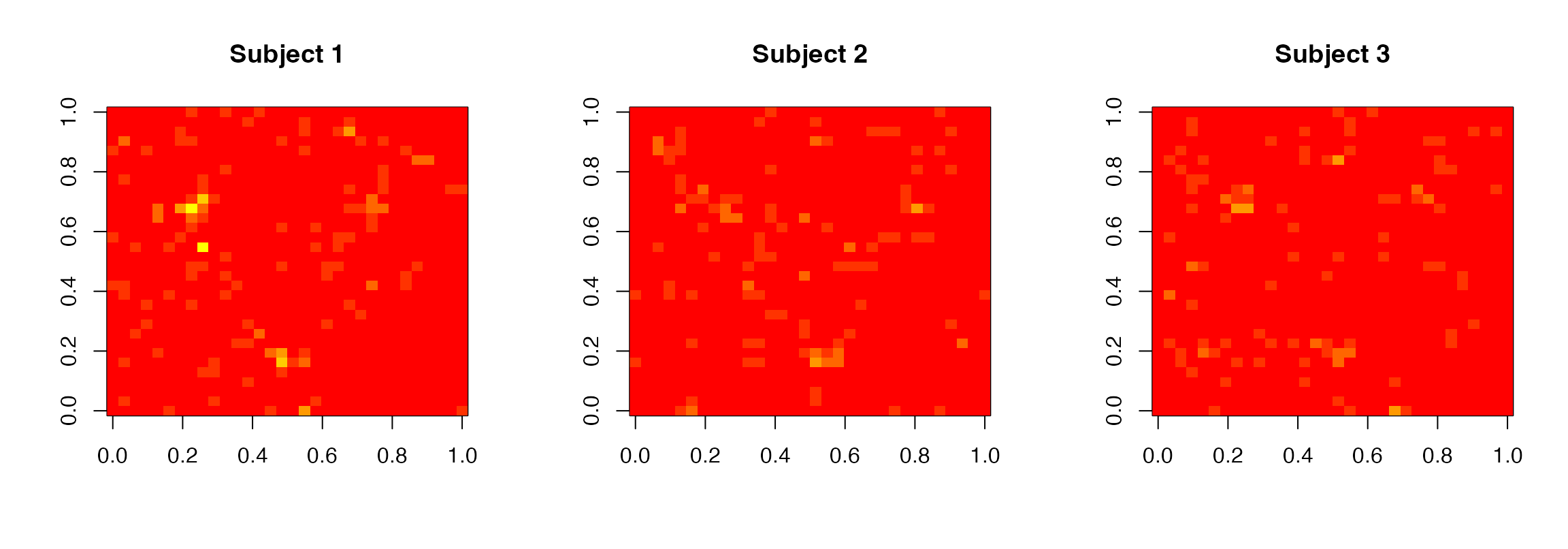Posterior standardized regression coefficient images for the 3 subjects obtained by BHMSMA Figure @ref(fig:PostCoef), obtained by the following code, shows the images of the posterior standardized regression coefficients for the 3 subjects. As the true coefficients are known, we can compute the mean squared error (MSE) using the following code. MSE <- c() for (i in 1:n) MSE[i] <- sum((as.vector(truecoef[i,,]/glmmap$GLMCoefSE[i,,,2])
- as.vector(postglmmap$GLMcoefposterior[i,,]))^2) round(MSE,3) ##  446.322 309.666 306.514 In (Sanyal and Ferreira 2012), we show that our multi-subject methodology performs better than some existing methodologies in terms of MSE. ### Posterior simulation and uncertainty estimation: postsamples In order to simulate observations from the posterior distribution of the regression coefficients, the function postsamples can be used. The type of analysis must be mentioned. The code below shows its use. Postsamp <- postsamples( nsample=50, n, grid, glmmap$GLMCoefStandardized[,,,k],
wavecoefglmmap$WaveletCoefficientMatrix, hyperest$hyperparam,
pkljbar$pkljbar, "multi", seed=123) names(Postsamp) ##  "samples" "postdiscovery" dim(Postsamp$samples)
##   3 32 32 50
dim(Postsamp$postdiscovery) ##  3 32 32 The argument nsample denotes the number of samples to be drawn. We can see postsamples returns the posterior samples and the probabilities of posterior discovery (Morris et al. 2011) for all the subjects. Figure @ref(fig:PostDiscovery), obtained by the following code, shows the posterior discovery images based on the above 50 samples for the 3 subjects. par(mfrow=c(1,n), cex=1) for(subject in 1:n) image(Postsamp$postdiscovery[subject,,], col=heat.colors(8),
main=paste0("Subject ",subject))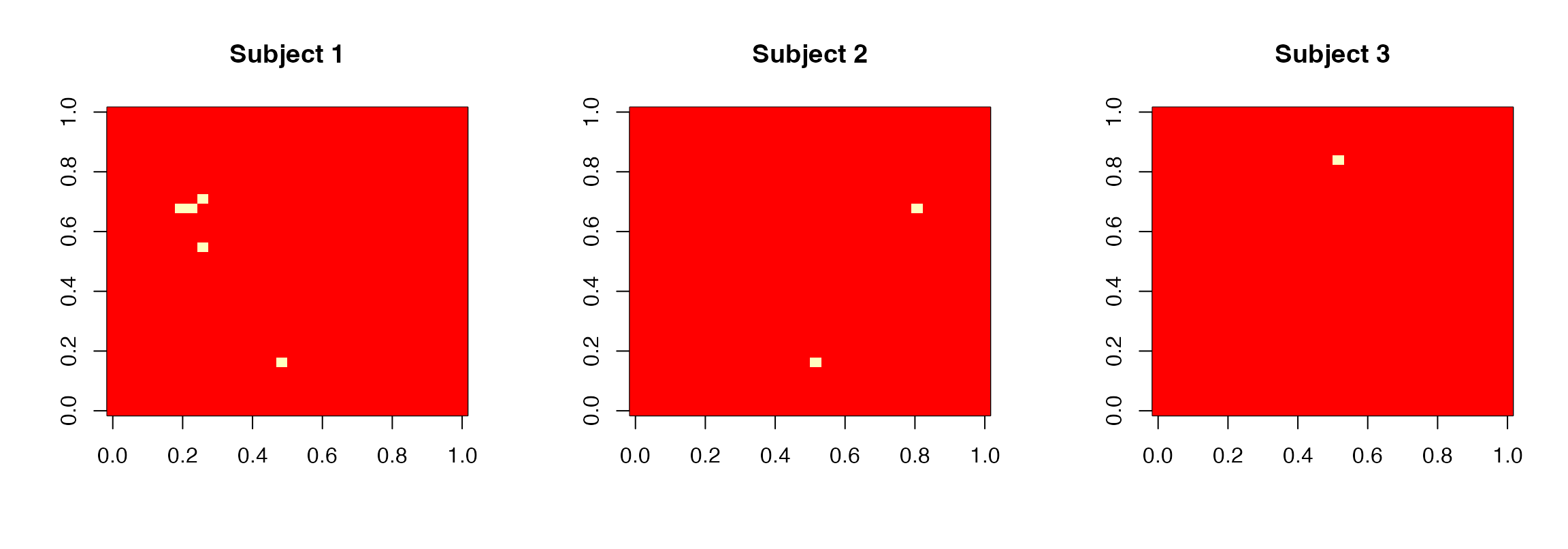Posterior discovery images for the 3 subjects

From the posterior samples, the posterior standard deviations of the regression coefficients can be computed as follows.

postsd <- array(dim=c(n,grid,grid))
for(subject in 1:n)
postsd[subject,,] <- apply(Postsamp$samples[subject,,,], 1:2, sd) round(postsd[1,1:5,1:5],3) ## [,1] [,2] [,3] [,4] [,5] ## [1,] 0.466 0.496 0.471 0.677 0.677 ## [2,] 0.655 0.737 0.531 0.677 0.687 ## [3,] 0.657 0.465 0.472 0.435 0.656 ## [4,] 0.656 0.702 0.470 0.581 0.597 ## [5,] 0.571 0.468 0.417 0.532 0.508 ### Posterior group estimates: postgroupglmcoef Posterior group coefficients can be obtained by using the function postgroupglmcoef as follows. postgroup <- postgroupglmcoef( n, grid, glmmap$GLMCoefStandardized[,,,k],
postwavecoefglmmap$PostMeanWaveletCoef) names(postgroup) ##  "groupcoef" dim(postgroup$groupcoef)
##  32 32

Figure @ref(fig:PostGroupCoef), obtained by the following code, shows the posterior group coefficient image for the simulated dataset.

par(mfrow=c(1,1),cex=0.5)
image(abs(postgroup\$groupcoef),col=heat.colors(8),zlim=c(0,6))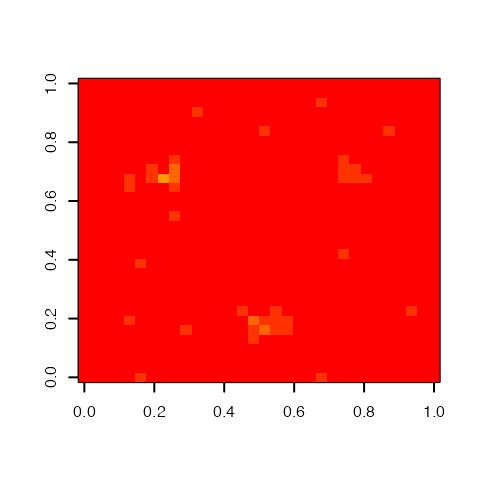Posterior group regression coefficient image

Cox, R. W. 1996. “AFNI: Software for Analysis and Visualization of Functional Magnetic Resonance Neuroimages.” Computers and Biomedical Research 29 (3): 162–73.
Friston, K. J., J. Ashburner, S. J. Kiebel, T. E. Nichols, and W. D. Penny, eds. 2007. Statistical Parametric Mapping: The Analysis of Functional Brain Images. Academic Press.
Goebel, R., F. Esposito, and E. Formisano. 2006. “Analysis of Functional Image Analysis Contest (FIAC) Data with BrainVoyager QX: From Single-Subject to Cortically Aligned Group General Linear Model Analysis and Self-Organizing Group Independent Component Analysis.” Human Brain Mapping 27 (5): 392–401.
Morris, J. S., V. Baladandayuthapani, R. C. Herrick, P. Sanna, and H. Gutstein. 2011. “Automated Analysis of Quantitative Image Data Using Isomorphic Functional Mixed Models, with Application to Proteomic Data.” Annals of Applied Statistics 5: 894–923.
Nason, Guy. 2013. “Wavethresh: Wavelets Statistics and Transforms.”
Sanyal, N., and M. A. R. Ferreira. 2012. “Bayesian Hierarchical Multi-Subject Multiscale Analysis of Functional MRI Data.” NeuroImage 63 (3): 1519–31.
Smith, S. M., M. Jenkinson, M. W. Woolrich, C. F. Beckmann, T. E. J. Behrens, H. Johansen-Berg, P. R. Bannister, et al. 2004. “Advances in Functional and Structural MR Image Analysis and Implementation as FSL.” NeuroImage 23 (SUPPL. 1): S208–19.
Welvaert, Marijke, Joke Durnez, Beatrijs Moerkerke, Geert Verdoolaege, and Yves Rosseel. 2011. neuRosim: An R Package for Generating fMRI Data.” Journal of Statistical Software 44 (10): 1–18.
Whitcher, Brandon, Volker J. Schmid, and Andrew Thornton. 2011. “Working with the DICOM and NIfTI Data Standards in R.” Journal of Statistical Software 44 (6): 1–28.